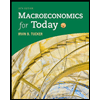Question

consider an economy in which the demand for money is of the form
Y / (1 + it)
for t = 0, 1, 2, · · · , where output is 150 and it denotes the nominal interest rate in period t. The real interest rate, denoted r, is constant and equal to 4%. In period
0 and 1, the money supply is 100 and people expect that money supply would
be 100 forever. People have rational expectations. In period 2, the central bank
surprises people and sets the money supply will grow at 2 percent forever, that is,
M0 = 100, M1 = 100, M2 = (1.02)M1, M3 = (1.02)M2, and so on.

A. Find the inflation rate, nominal interest rate, real money balance in period 1,
and expected inflation in period 2, given the information available in period
1, π1, i1, M1/P1  and, E1π2.

B. Find the inflation rate, nominal interest rate, real money balance in period 2, and expected inflation in period 3, given the information available in period
2, π2, i2, M2 / P2 and, E2π3.

C. Find the inflation rate, nominal interest rate, and real money balance in period 3, π3, i3, and M3 / P3
.

Expert Solution

### Want to see the full answer?

Check out a sample Q&A hereStudents who’ve seen this question also like:Not helpful? See similar booksMACROECONOMICS FOR TODAY
Monetary Policy. 1SQPWant to see this answer and more?
Experts are waiting 24/7 to provide step-by-step solutions in as fast as 30 minutes!*
*Response times may vary by subject and question complexity. Median response time is 34 minutes for paid subscribers and may be longer for promotional offers.